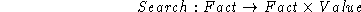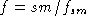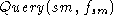Next: Update Up: Lazy Previous: Evaluation

### Search

Hence, given a query to a module, the algorithm sketched below shows how the module searches for the next question to be made to the user (Ask procedure) or to a submodule (Query procedure) that is needed to answer the current query. This algorithm returns only a fact belonging to the import interface of the current module, or a fact belonging to a submodule with its value.

This is a recursive algorithm because the initial goal produces new internal subgoals that in their turn use the same algorithm. Consider that f is the fact constituting the current query.1.is a path: If the fact is a path to a submodule sm of the current module, and if that submodule is visible (see Section 7), it puts a queryto the submodule and returns the value so obtained; otherwise it returns an error.
2. f has unsolved needs relations: The facts used in the body of the functional expression attached to f are considered as if f had a needs relation with them. Therefore, the needs relations of a fact are those explicitly declared by the expert plus those implicitly added by the function attribute. A needs relation is considered to be solved when the fact with which f has the relation got a value. It recursively applies Search to the first fact with which f has a no solved needs relation.
3. f belongs to the import interface and has no value: In this case it makes a question to the user Ask(f).
4. f can be deduced by means of rules:

1. Rule ordering: Before starting the rule search we order the set of rules that are able to deduce fact f with the following criteria:

1. Rules more specific first.
2. In case of rules equally specific, first those whose certainty value is higher.
3. In case of equal certainty degree, we use the writing order.

2. Rule search: We start a depth first search on the rulesby:

1. Selecting the first rule of the ordered set obtained above.
2. Selecting the first fact in the writing order of the conditions of that rule (left to right). Then recursively apply Search to that fact.

5. otherwise: f is unknown.

Notice that the algorithm Search finally puts a question to the user Ask(f), makes a query to a submoduleand returns the value obtained or, if everything fails it returns the value unknown.Next: Update Up: Lazy Previous: Evaluation

Josep Puyol-Gruart
Thu Oct 23 15:34:13 MET DST 1997